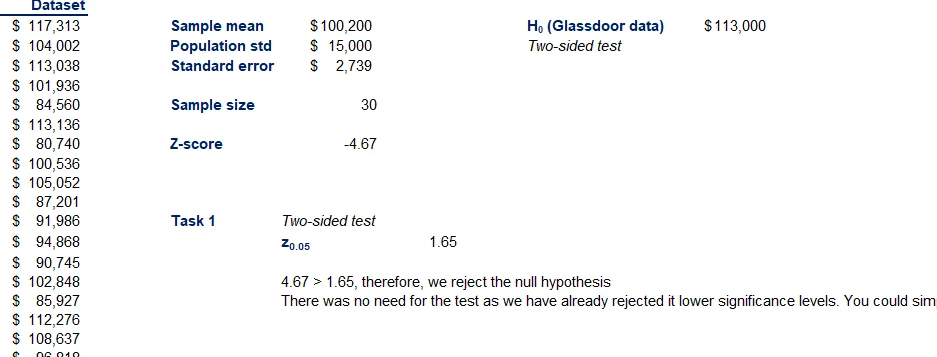06 Jan 2023

Posted on:

05 Jan 2023

0

# Resolved:Doubts in Exercise z Value

Exercise solution attached to this lesson I have doubts regarding the z value we find.
So we are finding 10% significance which means we have to find z(0.45) i.e alpha = 0.9 value whereas in exercise we are finding z(0.05) i.e alpha = 0.1 which is for 90% significance

z(0.45) = (-0.13)
z(0.05) = (1.65)

Z = -4.67 > -0.13 (although the Answer Remains the same that we reject Ho)Super learner
Posted on:

06 Jan 2023

0

The level of significance is denoted by alpha. If we want 10% significant level, we set alpha = 0.10.

Posted on:

06 Jan 2023

0

Lets see previously we take 99% or 95% significance alpha gone be 0.01 and 0.05 respectively so in that way for 10% significance alpha gone be 0.9

Super learner
Posted on:

06 Jan 2023

0

Hi Mayank,

I do not have the script of the lesson you are referring to, I simply guess that when you are talking about "previously we take 99% or 95% significance", are you referring to 99% (or 95%) confidence, instead of significance?

If it is about 99% confidence interval, then we take alpha = 0.01 (This is similar as 1% level of significance); and similarly, for 95% confidence, we take alpha = 0.05 (5% level of significance).

Hope this helps, and sorry if I am in the wrong direction in answering your query.